1. Back To Blog

# .NET interview questions: - How to parse data in Threading?

This is a semi-asked .NET interview questions and has been asked in most of the interviews to check your skills on parsing data in threading.

As a senior developer you would be interested to know how exactly we can parse data in threading.

Below is the code snippet for simple use of threading: -

```    class Program

static void Main(string[] args)

}
public static void CallMe()

for (int i = 0; i <= 5; i++)

}
}
}
```

In the above code snippet you can see that I have created a "CallMe" function which has a for loop and getting executed till the value of "i" is 5 and simply print a line of text to the output screen after every 2 seconds using Thread.Sleep(2000);.

Now, when you execute this above code snippet you will see result like below diagram.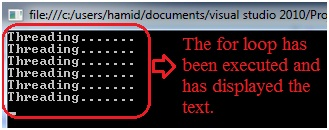The above code snippet seems to be works fine but what if there is a situation that you want to execute for loop according to the data passed by the Thread.

The data can be parsed in threading by the following two ways

1. You can parse the value by defining object variable.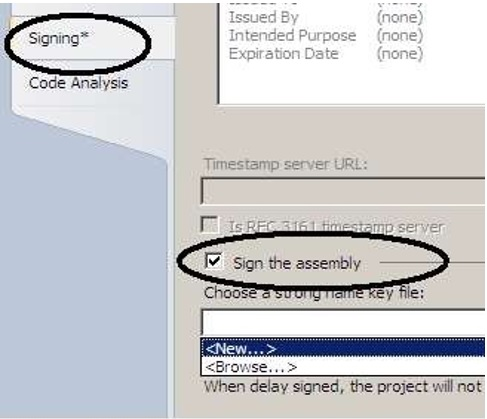2. You can use Lambda Expression.

So, let's take the above point's one by one try to understand it in better manner.

In order to see it practically you just need to follow the following steps.

Step1: - Create a simple Console Application for that just open Visual Studio >> go to >> File >> New >> Project >> Windows >> Select Console Application.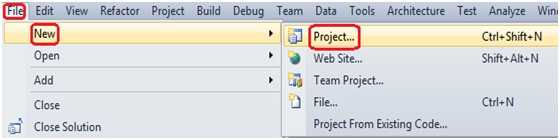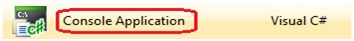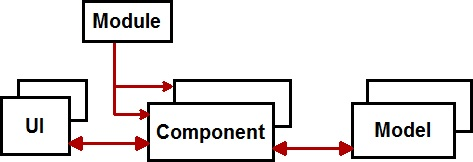Step2: - let us first prove the point of passing the data using object variables.

Now, simply just add the below code snippet in to program.cs file of your Console Application.

`   `
``` class Program

static void Main(string[] args)

//Passing the value 5.
}
//Created a CallMe function with a Object parameter "k".
public static void CallMe(object k)

//creted a int type variable and type caste it.
int j = (int)k;
for (int i = 0; i <= j; i++)

}
}
}
```

Now, just simply execute your console application and will see result like below diagram.This is how you can parse the data using Object Variables.

Step3: - similarly, let's take the second point of parsing the data using Lambda Expression.

Now, simply just change your program.cs file code snippet like below code snippet.

```    class Program

static void Main(string[] args)

//Created a Lambda Expression and parse the value as 7.
}
//Created a CallMe function with a int parameter "k".
public static void CallMe(int k)

//Created a int type variable which hold the value of k.
int j = k;
for (int i = 0; i < j; i++)

}
}
}
```

In the below diagram you can see that how I have used the Lambda Expression in order to parse the data in Threading.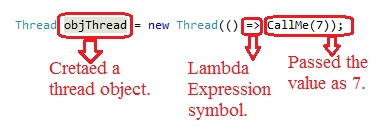Now, when you run your Console Application you will see the result like below diagram.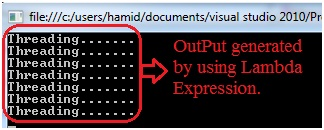Click for more  Dotnet interview questions and answers

Regards,

Visit for more author's blog on Most asked Dotnet interview questionsVisit us @ www.questpond.com or call us at 022-66752917... read more#### .NET interview questions 6th edition (Sixth edition) - By Shivprasad Koirala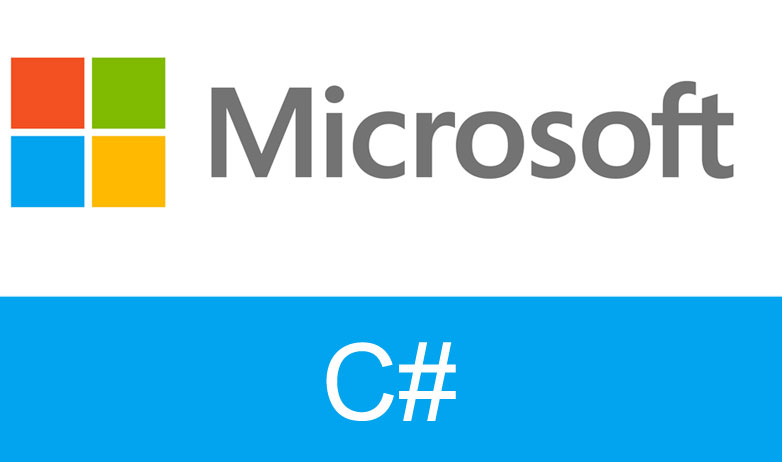#### C# Design pattern interview questions with answers: - How Singleton is different from Static class?#### Exception Handling in C# using example step by step#### MVC vs ASP.NET ( MVC Interview question with answers )?#### What is the difference between each version of MVC 2, 3, 4, 5 and 6? (MVC Interview Questions)